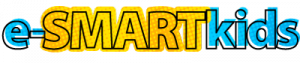# Investigate Your School’s Energy Habits Activity Tips

This advanced activity appears in the section Tell Me More – Energy Efficiency. The activity requires research and math skills.

Students will probably need you to walk them through the calculations. You can use the sample calculations below, which are based on estimates. Your school’s lighting costs, hours, number of lights, and electricity costs will vary from the example.

1. Choose a representative to speak with the head custodian about existing lights.
2. Contact a lighting store to find the most efficient lights to replace the existing ones. Find out:
• How much energy do new lights use? New lighting uses 50 watts for each 8-ft. fluorescent compared to 75 watts for old lighting. Electronic ballasts increase efficiency of the lighting system.
• How many hours do they last? Tubes last for approximately 20,000 hours of use.
• How much do they cost? Replacement cost = \$12 per tube and \$40 per fixture.
3. How many will be needed? 300 8-ft. tubes and 150 ballasts.
4. How long are the lights on per year? 1,400 hours/year.
5. Total watt-hours per year for both systems:Old system: 1,400 hours/year x 300 tubes x 75 watts = 31,500,000 watt-hours/year. This is the same as 31,500 kWh/year.New system: 1,400 hours/year x 300 tubes x 50 watts = 21,000,000 watt-hours/year. This is the same as 21,000 kWh/year.
6. Annual cost of running the different systems: If electricity cost per kWh is 10¢, then cost for each system is:Current system: 31,500 kWh/year x \$0.10/kWh = \$3,150/year

New system: 21,000 kWh/year x \$0.10/kWh = \$2,100/year

Savings from electronic ballast of 35%: \$2,100 x 0.35 = \$735

Total savings: kWh savings + electronic ballast savings = (\$3,150/year – \$2,100/year) + \$735/year = \$1,785/year

7. How long will it take to pay off the new lights?New system cost = (300 x \$12) + (150 x \$40) = \$3,600 + \$6,000 = \$9,600.

Consider that because some of the old tubes and ballasts would need replacement anyway, the additional cost of the new system would not actually be as high as this figure. If 1/3 of the tubes and ballasts needed replacing, then the real cost would be \$6,400. It would take about three and a half years for the energy savings to pay off the cost of the new equipment.

8. Share results with principal or custodian.
9. Other energy uses: energy for heating and air conditioning; electricity for classroom, office, and cafeteria equipment; fuel for school buses, etc.